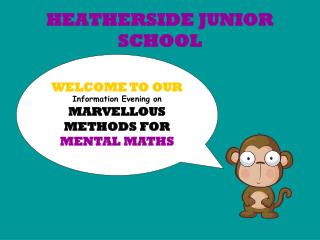DownloadDownload PresentationHEATHERSIDE JUNIOR SCHOOL

# HEATHERSIDE JUNIOR SCHOOL

Télécharger la présentation## HEATHERSIDE JUNIOR SCHOOL

- - - - - - - - - - - - - - - - - - - - - - - - - - - E N D - - - - - - - - - - - - - - - - - - - - - - - - - - -
##### Presentation Transcript

1. HEATHERSIDE JUNIOR SCHOOL WELCOME TO OURInformation Evening onMARVELLOUS METHODS FOR MENTAL MATHS

2. VOCABULARY • + Addition + • add • total • sum of • altogether • plus • increase by • double • more than • Subtraction – • subtract • take away • minus • count back / up • less than • fewer • find the difference • decrease by How do you know which type(s) of operation to use?

3. x Multiplication x • times • lots of • groups of • product of • multiplied by • multiple of • double • repeated addition • array • ÷ Division ÷ • share • share equally • equal groups of • divide • divided by • divided into • divisible by • remainder • factor • quotient • inverse

4. Progression in the use of number lines Firstly, children count using a number track. Then they use a numbered number line. Next children begin to use number lines with regular interval markings and some or no numbers marked. 64 70 Finally, they use an empty number line to record their jottings.

5. Children then use jottings to record numbers they have partitioned. (linked to using a number line) For example: 223 – 53 =170 223 – 50 – 3 =? 223 – 50 = 173 173 – 3 = 170

6. They can also use a number line to add and subtract by counting back and up using the nearest multiple of 10 and adjusting. For example: 36 – 19 = 17 - 20 16 17 36 + 1

7. Complements to 10 & 100 1 3 7 5 5 4 70 15 15 90 10 6 4 7.6 6.5 9.3 3.5 0.7 2.4 My number bonds will help here!

8. Quick recall games such as ‘Shoot the Sheriff’, FizzBuzz & Chase the answer Practice Games e.g bingo, pairs, snap Speedy Tables Race the clock and then aim to beat your score ! Fun ways to learn the times tables! Patterns and Rules of divisibility Do you know the trick your 9s? What do all multiples of 2 end in? Songs, raps and rhymes Practise, practise, do it again..practise a bit more…repeat, try again..practise..have another go.. Tools to help Tables squares, number lines, concrete apparatus, websites

9. By partitioning a number we can use known doubles of smaller numbers and then add these together to calculate the answer. Double 40 is relatively easy: 40 x 2 = 80 Double 7 is a known double: 7 x 2 = 14 Add these together  80 + 14 = 94 E.g. Double 47 is not a double that most people know off by heart.

10. Using known facts If I know that 6 x 4 = 24, what else do I know? How could I use my knowledge of the 2 times table to calculate the 4s or 8s? How could I use this to make some calculations with decimals? Eg 6 x 0.4 To calculate 17 x 6 I could do (10 x 6) + (7 x 6). How could I calculate 18 x 7? The keepers at the zoo want to know how many monkeys they have. There are 16 enclosures, each containing 8 monkeys. How many monkeys are there altogether? Use your knowledge of the 8, 4 or 2 times tables to calculate the answer.

11. Using inverse operations For every number operation (+ - x ÷) there is an inverse operation. For example: This can be used to calculate or check an answer: 7 x ? = 35 ? - 143 = 29 53 + 48 = 101 101 – 53 =48 101 – 48 = 53 12 x 3 = 36 36 ÷ 12 = 3 36 ÷ 3 = 12 ? + 45 = 110

12. Round up or round down? High 5 rule There are 150 children and teachers going on a theatre trip. They need to travel from the school to the theatre in coaches. Each coach can carry 60 children. How many coaches will be needed? 150 ÷ 60 = 2 r30 or 2.5 3 coaches needed Round up

13. What key facts/knowledge would you need to know to help you solve these questions? James walked from 09:45 to 10:15. For how many minutes did he walk? A regular hexagon has sides of 8cm. What is its perimeter? At the weekend I cycled 2.5km. My Dad cycled 200m further than me. How far did Dad cycle?

14. Mental maths relies on children being able to calculate quickly and accurately.

15. Please stay and have a go at some of the problems and activities…can you use some of the mental calculation strategies we have covered?